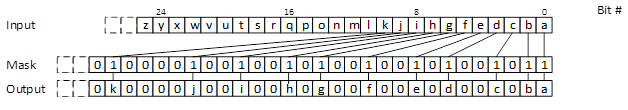# Resetting the Lowest n Set Bits

ID 761662
Updated 1/9/2017
Version Latest
PublicBy

Already a couple of years ago, the Bit Manipulation Instruction Set 1 (BMI1) introduced the instruction BLSR, which resets the lowest bit that is set. (The corresponding intrinsic _blsr_u32/64 wraps this instruction with some nice C/C++ function call syntax.) However, what are your options when you not only want to delete one bit, but a given number of bits n? This blog presents multiple variations of this theme including a performant implementation.A naïve implementation would step through the bits from lowest to highest. If a bit is set, it is reset this bit to 0 until the desired number of bits is reset:

__int64 blsrn_basic(__int64 a, int n) {
int i = 0;
while (i <= n) {
++i;
}
}
return a;
}


In fact, there is a little known instruction for testing and resetting a single bit: btr, which corresponds to the intrinsic _bittestandreset64. Using btr therefore simplifies the code as follows:

__int64 blrsn_btr(__int64 a, int n) {
int i = 0;
int pos = 0;
while (i <= n) {
if (_bittestandreset64((__int64*)&a, pos)) {
++i;
}
++pos;
}
return a;
}


A nice side-effect of this code change is that the compiler (Intel® Parallel Studio XE 2017 Update 1 Composer Edition for C++ in my case) is now smart enough to replace the branch inside the loop with a conditional move. This avoids a branch that is hard to predict as it depends on the input data.

Nevertheless, it is annoying that we need to loop through all the zeros, when we are only interested in the ones. The instruction trailing zero count TZCNT can help here, in that TZCNT directly tells us the position for the lowest bit that is set:

__int64 blrsn_tzcnt(__int64 a, int n) {
for (int i = 0; i <= n; ++i) {
__int64 mask = 1ULL << _tzcnt_u64(a);
}
return a;
}


Attentive readers might have observed already, that it is not necessary to determine the position of the lowest bit set. As mentioned in the introduction, the blsr instruction simply resets the lowest bit set. It is therefore sufficient, to call _blsr_u64 for n times:

__int64 blrsn_blsr(__int64 a, int n) {
for (int i = 0; i <= n; ++i) {
a = _blsr_u64(a);
}
return a;
}


This code is obviously way more effective than the naïve implementation that we started with. On my Intel® Core™ i5-4300U processor I measures about a factor of 8 for some random input data, but – as always – your mileage way vary with processor, compiler, and test data.

However, even with this code, the problem remains that we are resetting single bits. Furthermore, the loop has an unpredictable trip count as it depends on the input n, the number of bits that should be reset. Wouldn’t it be nice if we can remove this loop altogether? In order to achieve this, the instruction PDEP (with intrinsic _pdep_u64) from BMI2 can be of great help. PDEP deposits (scatters) bits from the input value to the output value according to a given mask:If we use as input a value with all ones, except for the n lowest bits, this has the same effect as resetting n lowest bits in the mask:Furthermore, such an input with n trailing zeros can be realized with the instruction bzhi, which clears the upper bits of its argument. By negating the result, we obtain the desired input for pdep. The resulting code therefore condenses to the following two lines, which completely remove the loop:

__int64 blrsn_pdep(__int64 a, int n) {